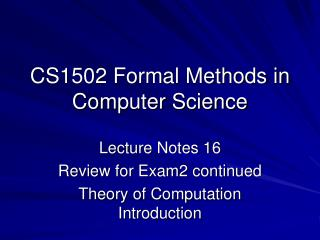Download PresentationCS1502 Formal Methods in Computer Science

CS1502 Formal Methods in Computer Science - PowerPoint PPT Presentation

CS1502 Formal Methods in Computer Science. Lecture Notes 16 Review for Exam2 continued Theory of Computation Introduction. 10.25. Is the following a logical truth? ~exist z Small(z) ïƒŸïƒ  exist z ~Small(z). 11.16. 1. Every cube is to the left of every tet.I am the owner, or an agent authorized to act on behalf of the owner, of the copyrighted work described.
Download PresentationCS1502 Formal Methods in Computer Science

Download Policy: Content on the Website is provided to you AS IS for your information and personal use and may not be sold / licensed / shared on other websites without getting consent from its author.While downloading, if for some reason you are not able to download a presentation, the publisher may have deleted the file from their server.

- - - - - - - - - - - - - - - - - - - - - - - - - - E N D - - - - - - - - - - - - - - - - - - - - - - - - - -
Presentation Transcript
1. CS1502 Formal Methods in Computer Science Lecture Notes 16 Review for Exam2 continued Theory of Computation Introduction

2. 10.25 • Is the following a logical truth? ~exist z Small(z)  exist z ~Small(z)

3. 11.16 1. Every cube is to the left of every tet. 2. Every small cube is in back of a large cube. 3. Some cube is in front of every tet. 4. A large cube is in front of a small cube.

4. 11.16 5. Nothing is larger than everything which is 6. Every cube in front of every tet is large. 7. Everything to the right of a large cube is small. 8. Nothing in back of a cube and in front of a cube is large.

5. 11.16 9. Anything with nothing in back of it is a cube. 10. Every dodec is smaller than some tet.

6. 11.20 1. Nothing to the left of a is larger than everything to the left of b 3. The same things are left of a as are left of b.

7. 11.20 7. Only dodecs are larger than everything else. Answer in solution: Is this correct? Hmm….

8. Theory of Computation • Sipser text covers automata, computability, and complexity • CS1502: automata (chapter 1) • CS1511 uses the same text

9. Theory of Computation • What are the fundamental capabilities and limitations of computers?

10. Complexity Theory • Classes of problem difficulty (sorting < sched) • The **problems**, not the code • If can show a problem is in a certain class: • ‘easy’ keep working on an efficient solution! • ‘hard’ give up, you won’t find a fast solution • Perhaps modify the problem into an easier one; maybe solving that is good enough for what’s needed • Cryptography – want problem so decoding is intractable • Still important/answered questions. As new models of computation arise: what is tractable/intractable?

11. Computability Theory • Which problems can be solved on a computer? • Turing Machine: • Abstract away from particular hardware, OS, PL, algorithm • Can compute anything that is a Turing machine

12. Automata Theory • Begins with the question, what is a computer? • Real computers are too complex and messy to model. So, we define computational models (idealized computer)

13. Finite Automata; Finite-State Machines • Simplest machines • Cannot compute everything,but operations are cheap and efficient. So, use them if you can! • Used in text processing, compilers, hardware design, etc. • Composed of states and transitions between states